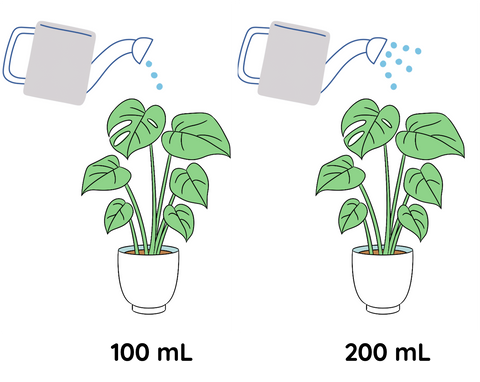# Independent, Dependent and Controlled Variables

This is part of the NSW HSC science curriculum part of the Working Scientifically skills.

### What are Independent, Dependent, and Controlled Variables?

As a high school science student, you are likely to come across different types of variables in your experiments. Being able to recognise these variables is a skill which is included in the NSW Higher School Certificate (HSC) curriculum. These variables are essential to scientific inquiry as they help us understand how different factors affect the outcomes of experiments. There are three main types of variables in scientific investigations: independent, dependent, and controlled variables. We will explore each of these variables and their importance in scientific inquiry.

### Independent Variables

Independent variables are the variables that are manipulated or changed by the researcher in an experiment. They are also known as the input variables or the cause variables because they are the factors that cause changes in the dependent variable.

For example, if you were investigating the effect of temperature on the rate of photosynthesis in plants, temperature would be the independent variable. You would manipulate the temperature to see how it affects the rate of photosynthesis.

It is essential to note that an experiment should have only one independent variable. This is because if you change more than one variable, you will not know which variable caused the change in the dependent variable. Therefore, by controlling the independent variable, you can determine the effect of that variable on the dependent variable.

### Dependent variables

Dependent variables are the variables that are affected by the independent variable in an experiment. They are also known as the outcome variables or the effect variables. The dependent variable is what you measure or observe to determine the effect of the independent variable.

For example, in the temperature and photosynthesis experiment, the dependent variable would be the rate of photosynthesis, which is affected by changes in temperature.

It is crucial to keep the dependent variable constant during an experiment to ensure that any changes observed are a result of changes in the independent variable. Additionally, the dependent variable should be measurable and quantitative, meaning that it can be expressed in numerical values.

### Controlled variables

Controlled variables are the variables that are kept constant during an experiment to ensure that they do not affect the outcome. These variables are also known as constant variables or the controlled factors. The purpose of controlling these variables is to ensure that any changes observed in the dependent variable are due to changes in the independent variable and not due to other factors.

For example, in the temperature and photosynthesis experiment, the controlled variables would include factors such as the type of plant, the amount of light, and the amount of carbon dioxide. By keeping these variables constant, you can ensure that any changes in the rate of photosynthesis are due to changes in temperature and not due to other factors.

### Identifying variables

Let's consider a scenario where we want to investigate the effect of different amounts of water on plant growth. In this case:1. Independent variable: The independent variable in this experiment is the amount of water used to water the plants. We could use different amounts of water, such as 100 ml, 200 ml, or 300 ml.

2. Dependent variable: The dependent variable is still the growth of the plants, which we could measure by tracking the height, weight, or number of leaves of the plants.

3. Controlled variables: Some controlled variables in this experiment might include the type and species of plants used, the type and amount of soil used, the size and type of pots used, and the amount of sunlight and temperature that the plants are exposed to.

By identifying and controlling these variables, we can design a more controlled and rigorous experiment to investigate the effect of different amounts of water on plant growth.